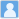• ### Exact Decomposition of Multifrequency Discrete Real and Complex Signals

分类： 电子与通信技术 >> 信息处理技术 分类： 工程与技术科学 >> 工程通用技术 分类： 机械工程 >> 机械工程其他学科 提交时间： 2022-02-08刘保国

‘The spectral leakage (SL) from windowing and the picket fence effect (PEF) from discretization’ have been among the standard contents in textbooks for many decades. The SL and PEF would cause the distortions in amplitude, frequency, and phase of signals, which have always been of concern, and attempts have been made to solve them. This paper proposes two novel decomposition theorems that can totally eliminate the SL and PEF, they could broaden the knowledge of signal processing. First, two generalized eigenvalue equations are constructed for multifrequency discrete real signals and complex signals. The two decomposition theorems are then proved. On these bases, exact decomposition methods for real and complex signals are proposed. For a noise-free multifrequency real signal with m sinusoidal components, the frequency, amplitude, and phase of each component can be exactly calculated by using just 4m–1 discrete values and its second-order derivatives. For a multifrequency complex signal, only 2m–1 discrete values and its first-order derivatives are needed. The numerical experiments show that the proposed methods have very high resolution, and the sampling rate does not necessarily obey the Nyquist sampling theorem. With noisy signals, the proposed methods have extraordinary accuracy.

同行评议状态:待评议